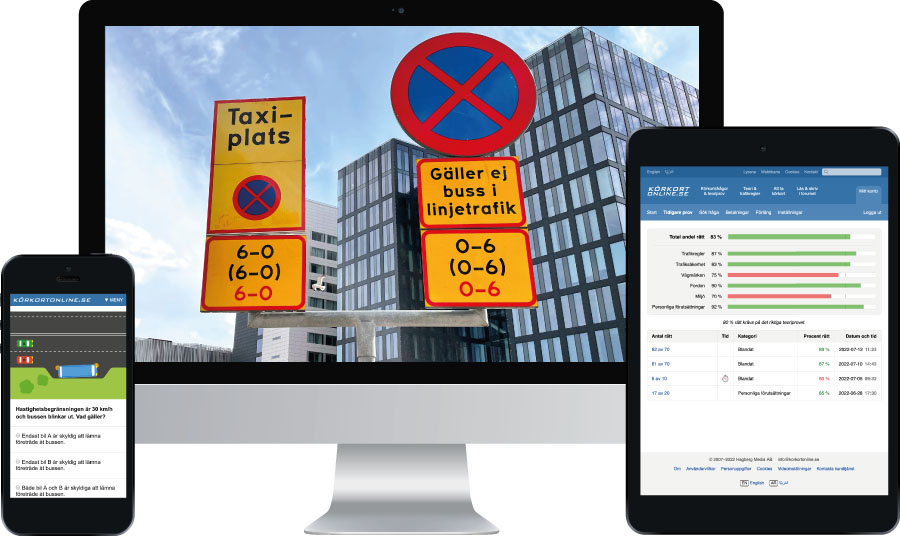# Driving theory questions

This is an old question that is no longer in use.

Images may be missing and the information may be dated.

Go to the current theory test questions (2023) >>

You are travelling at 30 km/h. How long will the braking distance be, if it is 41 metres at 60 km/h?

The braking distance [...] increases quadratically with increasing speed.” (Driving Licence Book, 19th Edition, page 120)

 Colour codes for the figures (easier to keep track of them) Current speed 30 km/h Comparative speed 60 km/h Comparative braking distance 41 metres Reduction in speed (to be calculated) X times Reduction in braking distance (to be calculated) X times

The answer is arrived at in several steps. The first question to ask yourself is whether the speed is increasing or decreasing. It is decreasing (from 60 to 30). But what we need to know is by how much it decreases:

• 60 / 30 = decreases by 2 times

The next step is to find out how much shorter the braking distance will be:

• 22 = 4 times shorter (22 is the same as 2 * 2)

To the power of 2 as the braking distance increases quadratically with the speed. The term “quadratically” corresponds to the meaning of “to the power of 2”.

We now know that the braking distance is 4 times shorter. In order to arrive at the distance in metres (as the question is asking for), the following calculation is used:

• 41 / 4 = approximately 10 metres.

Note that the road is probably very slippery, as 10 metres at 30 km/h is a fairly long braking distance.

Körkortonline.se offers driving licence theory tests. This is an example of an explanation of a test question. English theory test (free demo), Swedish theory test (free demo) and Arabic theory test (free demo).

More examples of explanations:

Category:

## Buy all 1000 questions in EnglishPersonal account with all 1000 questions.

Unlimited tests during the validity period.

Choose validity period: